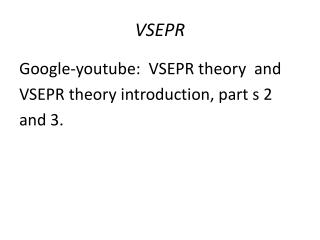# VSEPR - PowerPoint PPT PresentationDownload PresentationVSEPR

VSEPRDownload Presentation## VSEPR

- - - - - - - - - - - - - - - - - - - - - - - - - - - E N D - - - - - - - - - - - - - - - - - - - - - - - - - - -
##### Presentation Transcript

1. VSEPR Google-youtube: VSEPR theory and VSEPR theory introduction, part s 2 and 3.

2. VSEPR Bonding electrons + lone pairs =2 Electron Geometry = Linear MolecularGeometry = Linear

3. VSEPR Bonding electrons + lone pairs =3 Electron Geometry = Trigonal Planar Molecular Geometry (BH3 = Trigonal Planar) (SO2 = Bent)

4. VSEPR Bonding electrons + lone pairs =4 Electron Geometry = Tetrahedral Molecular Geometry : (CH4 =tetrahedral) ( NH3 = Trigonalprymidal) Molecular Geometry: (H2O = bent)

5. VSEPR Bonding electrons + lone pairs =5 Electron Geometry = Trigonalbipyramidal Molecular Geometry : (PCl5 = Trigonalbipyramidal) (SF4 = See Saw) Molecular Geometry : (ClF3 = T-shaped) (I3- = Linear)

6. VSEPR Bonding electrons + lone pairs =6 Electron Geometry = Trigonalbipyramidal Molecular Geometry : (PCl5 = Trigonalbipyramidal) (SF4 = See Saw) Molecular Geometry : (ClF3 = T-shaped) (I3- = Linear)

7. VSEPR Bonding electrons + lone pairs =6 Electron Geometry = Octahedral Molecular Geometry : (SF6 = Octahedral) (BrF5 = Square Pyramidal) Molecular Geometry : (XeF4 = Square planar)

8. VSEPR Steric No. = #bonds + #lone pairs

9. VSEPR PROBLEMSPredict the electron geometry, molecular geometry, and bond angles for the following: • C2H2 2. XeF2 3. C2H2 4.CH2O 5. I3- 6. IF2- 7. NO2- 8. IF4- 9. IF4+ • CO32- 11. HCN 12. IF5 13. PF6- 14. CH2O2 15. CH3COCH3